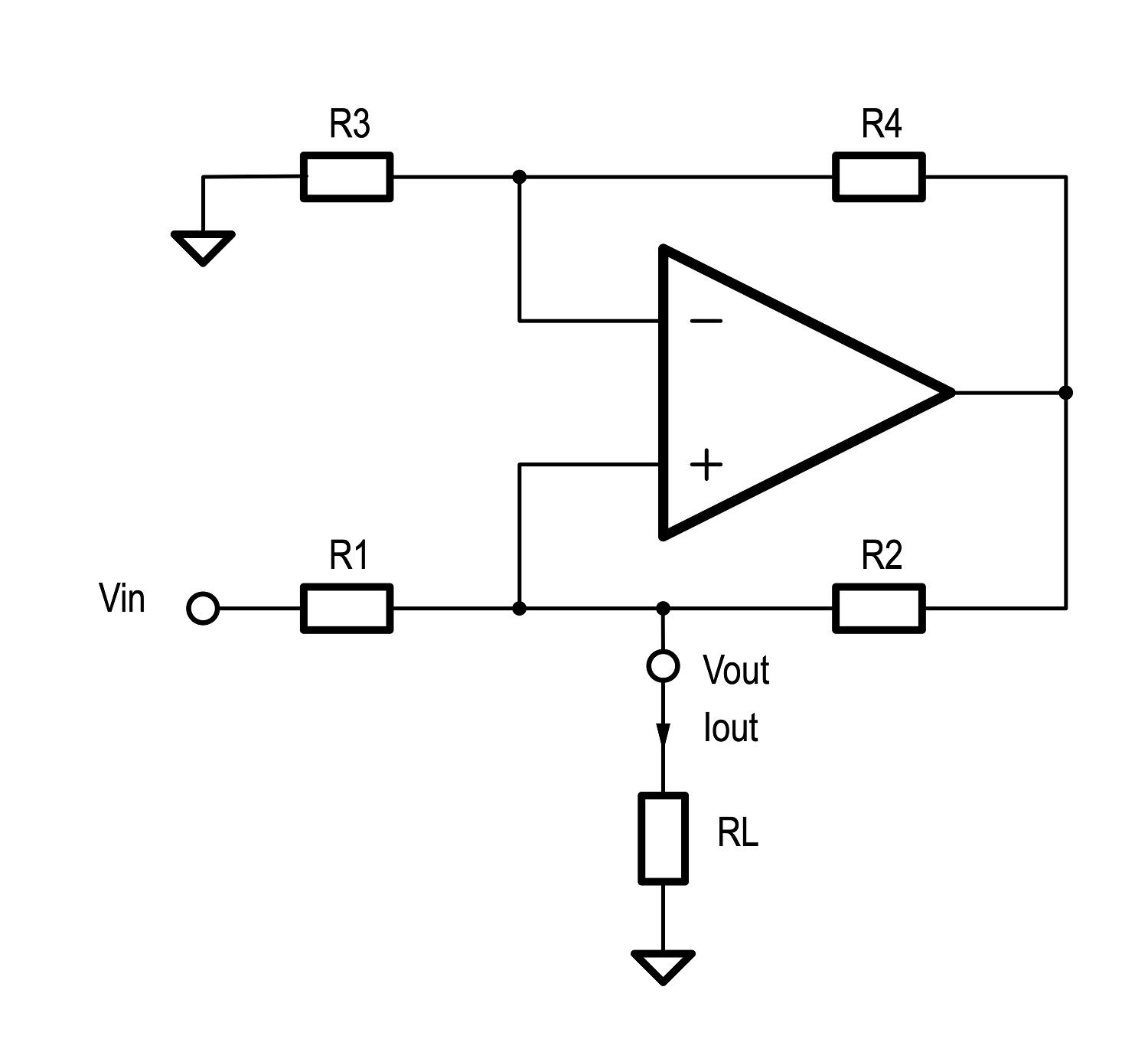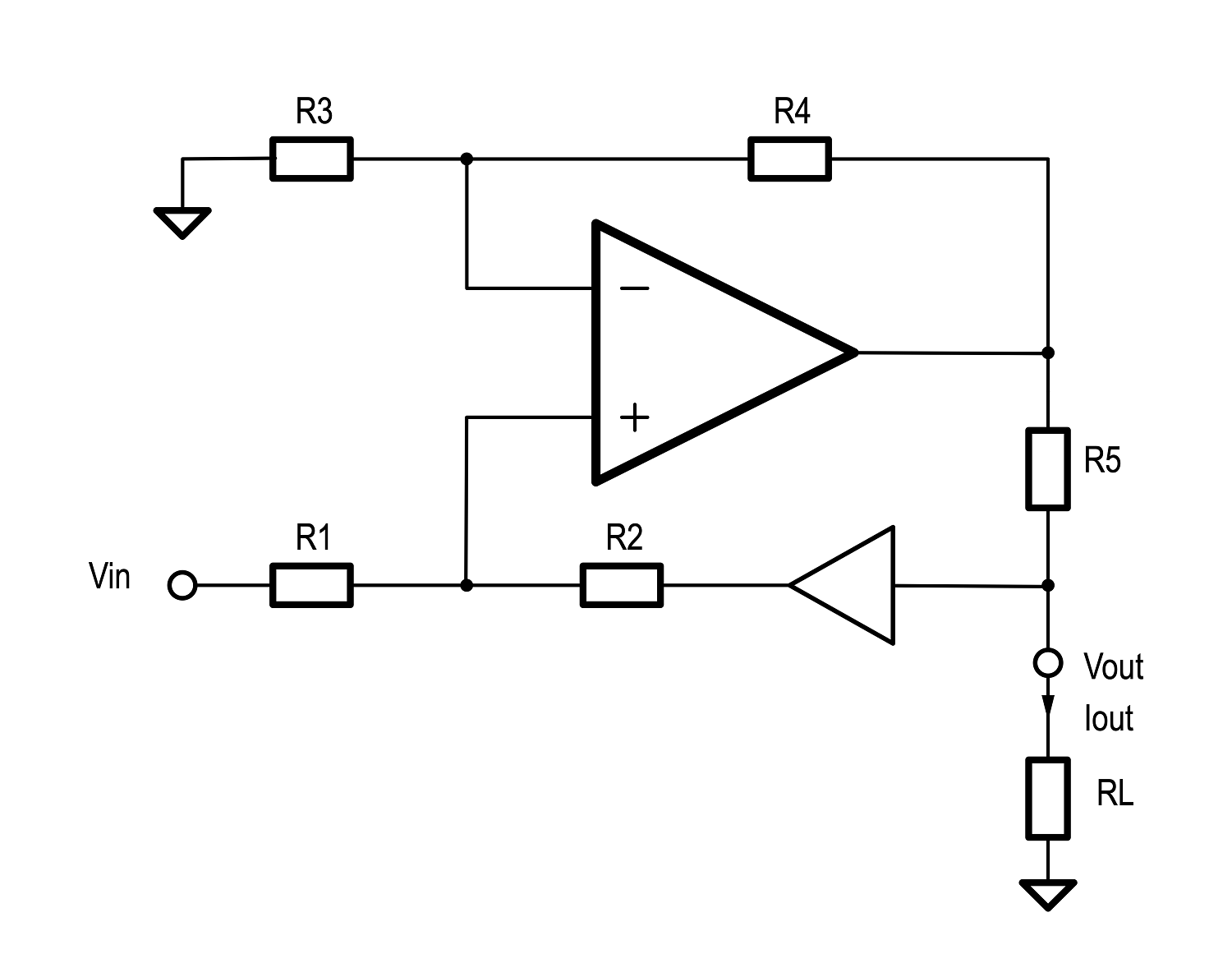# Howland Current SourceWritten by

A voltage controlled current source is a very useful circuit building block, and the Howland current source is a good example. Devised by Professor Bradford Howland at MIT in the early 1960s, it is capable of both sourcing and sinking current proportional to an input voltage. It is sometimes also called a Howland current pump.FIGURE 1. The basic Howland current source circuit looks a lot like a differential amplifier, except the output node is at the non-inverting input of the op amp. The current flowing out of this node through the load is the sum of the currents entering the node via R1 and R2.

Figure 1 shows the basic configuration. The current flowing through the load resistor is the sum of the currents through the resistors R1 and R2. We can also see that the op amp forms a non-inverting amplifier. This gives us two equations:

Substituting the second into the first gives the expression:

Note that the expression in brackets is in inverse units of resistance and is effectively the output impedance of the circuit as seen from the output node. Let’s call this R0. Now we have:

If we set the ratio of R1/R2 equal to the ratio of R3/R4 (for example by making all these resistances the same), the impedance of the output node will tend towards infinity and the output will therefore be a voltage controlled current source with value of vi/R1

In reality, you do have to have well matched resistor ratios and an op amp with good CMRR in order to get the best out of the circuit. The references below suggest some techniques for trimming out any mismatch.

Table 1 shows some values as an example, with all for resistors set to 1kΩ and the input voltage set to 2V. For different values of output voltage (and therefore different load resistances) we can see that the output current remains constant.

Like all circuits, there are limitations – especially in the output voltage swing. If the resistor ratios are 1:1 the swing is limited to less than half of the op amp supply voltage. You can improve this a bit with careful selection of the resistor values but can introduce other problems.FIGURE 2. This enhancement to the Howland source allows the output node to swing closer to the supply rails. It does however introduce a requirement that R5 be much smaller than R2 to avoid unnecessary error.

Figure 2 shows an enhanced version of the Howland current source. In this case the output current can be shown to be:

Now we can set R5 to be quite a low value and take advantage of a much greater output swing.  However, there is a compromise (as there almost always is). In order for this circuit to work you need to satisfy two conflicting requirements. Not only does R1/R2 and R3/R4 have to be equal to k, we also need R1/(R2 + R5) to be equal to k as well. If we choose R5 to be much smaller that R2, we can approximate this, but it is not perfect.FIGURE 3. Introducing a unity-gain buffer between the output node and R2 decouples the R5 and R2 eliminating the requirement that R5 be much smaller in value than R2 that we have in Figure 2.

A solution is to add a buffer stage as shown in Figure 3. This effectively decouples R5 and R2, eliminating the need to satisfy the equation containing R5 above, allowing us freedom to choose the value of R5 independently.

References:

“A Large Current Source with High Accuracy and Fast Settling | Analog Devices.” Accessed April 7, 2021. https://www.analog.com/en/analog-dialogue/articles/a-large-current-source-with-high-accuracy-and-fast-settling.html.

“AN-1515 A Comprehensive Study of the Howland Current Pump.” Texas Instruments, April 2013. https://www.ti.com/lit/an/snoa474a/snoa474a.pdf.

 Keep up-to-date with our FREE Weekly Newsletter! Don't miss out on upcoming issues of Circuit Cellar. Subscribe to Circuit Cellar Magazine Note: We’ve made the May 2020 issue of Circuit Cellar available as a free sample issue. In it, you’ll find a rich variety of the kinds of articles and information that exemplify a typical issue of the current magazine. Would you like to write for Circuit Cellar? We are always accepting articles/posts from the technical community. Get in touch with us and let's discuss your ideas.